FX168财经网>人物频道>帖子

## 美林(Merrill)形态

• 概述
• 定义与应用
• 开发测试工具
• 结束语

### 概述

1971 年，罗伯特·利维（Robert Levy）首次尝试建立了价格形态系统。 他应用了五点价格波动形态，然后检查它们是否有意义。 他没有达成任何显著绩效，但是 10 年后，亚瑟·美林（Arthur Merrill）继承了他的工作。

• 上行趋势
• 下行趋势
• 三角形
• 扩展
• 头顶肩
• 逆头顶肩

### 定义与应用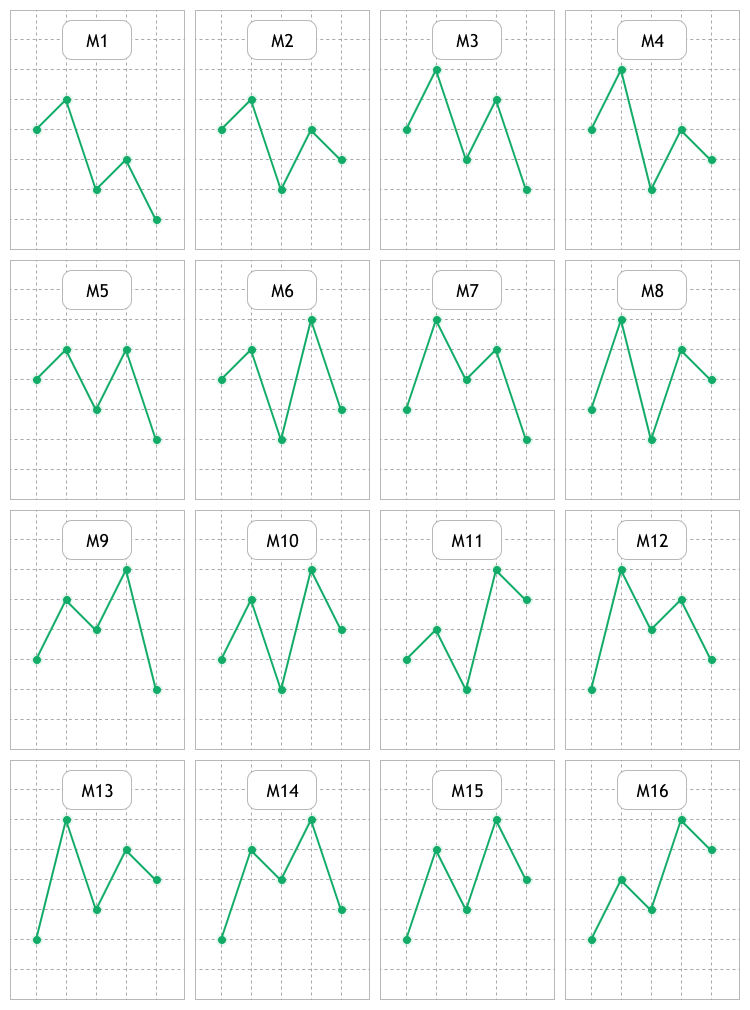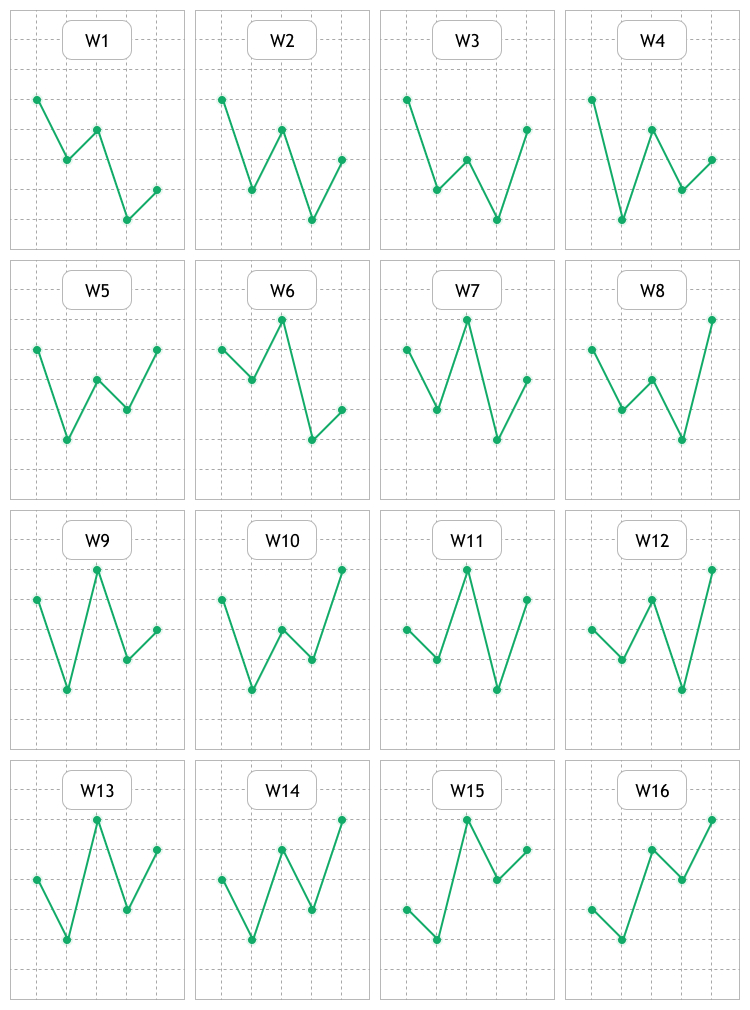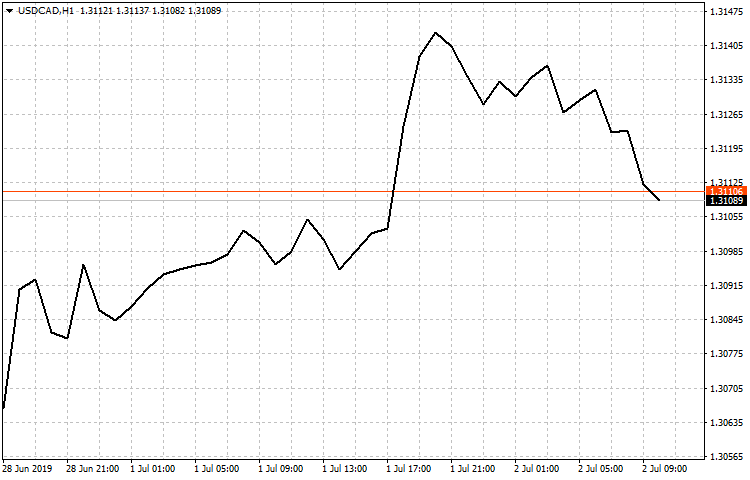• 平均真实范围 (ATR) — 市场波动率参数。
• 商品通道指数 (CCI) 衡量品种价格与其平均价格的偏差。
• DeMarker (DeM)。
• 推动力指数 (FRC)。
• 威廉姆斯的百分比范围 (WPR) — 定义超买/超卖状态的动态指标。
• 相对强度指数 (RSI)。
• 动量 — 给定时间范围内金融产品价格的变化。

• 在指定样本部分识别所分析形态。
• 识别后再分析价格走势。
• 收集数据并计算形态的效能。

### 开发测试工具

1. 两组按钮，用于选择测试形态的类型。 还有 All MAll W 按钮，用来快速选择/取消选择 М 和 W 形态。
2. 用于选择测试时间帧的按钮组，和用于选择/取消选择整个按钮组的 ALL 按钮。
3. “趋势阈值（点数）”输入字段。 这是在识别出所分析的美林形态后，价格应在最多三根蜡烛内达到的盈利点数。
4. 打开对话框窗口的按钮，用于选择开始和结束日期，以及测试时间。
5. 带有复选框和按钮的输入字段则是用来搜索必要交易品种的过滤器。 它已有个预设 — Major（主要）。 它显示主要货币对。 复选框则禁用过滤器，并显示所有可用的交易品种。
6. 在表格中配合过滤器选择交易代码。 从列表中选出它们之后，将执行形态分析。
7. 结果表格由七列组成：
• 图形名称。 该列显示所分析的美林形态名称，例如 M10 或 W12。
• 发现。 按所选样本检测到的指定类型的形态数量。
• 时间帧。 在时间帧内分析指定形态。
• P, 上行趋势。 形态出现后，价格可能向上移动的“趋势阈值（点数）”值。
• P, 下行趋势。 形态出现后，价格可能向下移动的“趋势阈值（点数）”值。
• K, 上行趋势/K, 上行趋势。 这个比率在我的文章研究烛台分析技术（第一部分）：检查现有形态中已有所阐述。 它评估所分析形态在趋势向上或向下方向出现之后，价格多快达到指定的利润。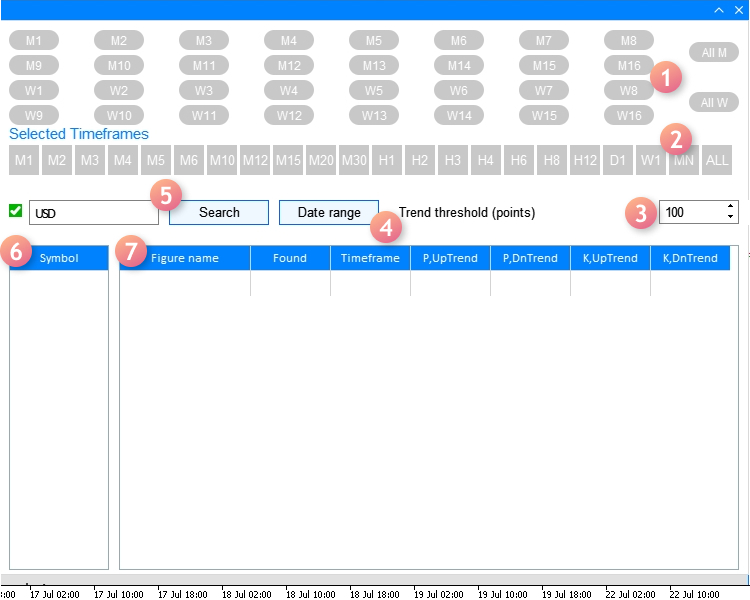1. 用到的指标。 选择一个指标，搜索并分析所应用的美林形态。
2. 在计算上述 K,上行趋势/下行趋势的比率时用到的权重系数。
3. 界面语言。 选择界面语言的下拉列表：英语或俄语。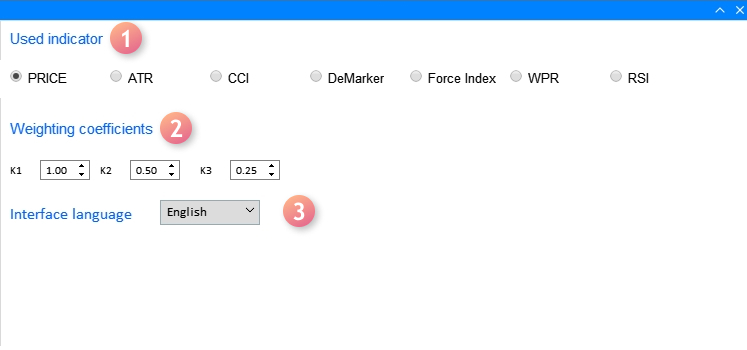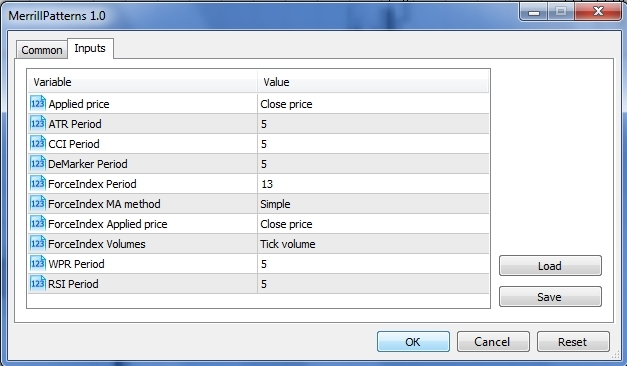• 第一个（“应用价格”）应用 ENUM_APPLIED_PRICE 枚举类型变量，该变量有七个值：开盘价，收盘价，最高价和最低价，以及中间价，典型价格和加权平均价格。 在基于图表价格执行分析时，应使用前四个值，因为后三个是为指标计算而设计的。
• 如果要在分析形态时使用指标，某些指标在计算时会将“应用价格”设置为 ENUM_APPLIED_PRICE 类型变量，即：ATR，CCI 和 RSI。

```//+------------------------------------------------------------------+
//| Create the program GUI                                           |
//+------------------------------------------------------------------+
bool CProgram::CreateGUI(void)
{
//--- Create the panel
if(!CreateWindow("Merrill Patterns"))
return(false);
//--- Create the dialog window
if(!CreateWindowSetting1("Setting dates"))
return(false);
//--- Complete GUI creation
CWndEvents::CompletedGUI();
return(true);
}
```

```//+------------------------------------------------------------------+
//| Analyze tab                                                      |
//+------------------------------------------------------------------+
//--- Create the pattern set buttons
if(!CreatePatternSet(m_patterns,10,10))
return(false);
if(!CreateTFLabel(m_text_labels,10,105,0))
return(false);
//--- Create the timeframe set buttons
if(!CreateTimeframeSet(m_timeframes,10,125,0))
return(false);
//--- Field for searching the symbol filter
if(!CreateSymbolsFilter(m_symb_filter1,m_request1,10,180,0))
return(false);
//--- Create the button for selecting a date range
if(!CreateDateRange(m_request3,280,180,0))
return(false);
//--- Create the field for entering the profit threshold value
if(!CreateThresholdValue(m_threshold1,400,180,100,0))
return(false);
//--- Create the symbol table
if(!CreateSymbTable(m_symb_table1,10,225,0))
return(false);
//--- Create the result table
if(!CreateTable1(m_table1,120,225,0))
return(false);
```

```//+------------------------------------------------------------------+
//| Settings tab                                                     |
//+------------------------------------------------------------------+
//---
if(!CreateButtonsGroup1(10,50))
return(false);
//--- Text labels
if(!CreateTextLabel(m_text_labels,10,100))
return(false);
if(!CreateTextLabel(m_text_labels,10,10))
return(false);
//--- Input fields
if(!CreateCoef(m_coef1,10,140,"K1",1))
return(false);
if(!CreateCoef(m_coef2,100,140,"K2",0.5))
return(false);
if(!CreateCoef(m_coef3,200,140,"K3",0.25))
return(false);
if(!CreateLanguageSetting(m_lang_setting,10,180,1))
return(false);
//--- Status bar
if(!CreateStatusBar(1,26))
return(false);
//---
return(true);
}
```

```//+---------------------------------------------------------------------------------+
//| Create the dialog window for selecting the range of dates in the Analysis tab   |
//+---------------------------------------------------------------------------------+
bool CProgram::CreateWindowSetting1(const string caption_text)
{
//--- Add the window pointer to the window array
//--- Coordinates
int x=m_request3.X();
int y=m_request3.Y()+m_request3.YSize();
//--- Properties
m_window.XSize(372);
m_window.YSize(230);
m_window.WindowType(W_DIALOG);

//--- Create the form
if(!m_window.CreateWindow(m_chart_id,m_subwin,caption_text,x,y))
return(false);
//---
if(!CreateCalendar(m_calendar1,m_window,10,25,D'01.01.2019',1))
return(false);
if(!CreateCalendar(m_calendar2,m_window,201,25,m_calendar2.Today(),1))
return(false);
//---
if(!CreateTimeEdit(m_time_edit1,m_window,10,200,"Time",1))
return(false);
if(!CreateTimeEdit(m_time_edit2,m_window,200,200,"Time",1))
return(false);
//---
return(true);
}
```

```//--- Include the application class
#include "Program.mqh"
CProgram program;
//+------------------------------------------------------------------+
//| EA inputs                                                        |
//+------------------------------------------------------------------+
input ENUM_APPLIED_PRICE   Inp_Price1              =  PRICE_CLOSE;   // Applied price
input int                  Inp_ATR_Peroid          =  5;             // ATR Period
input int                  Inp_CCI_Peroid          =  5;             // CCI Period
input int                  Inp_DeM_Peroid          =  5;             // DeMarker Period
input int                  Inp_ForcePeriod         =  13;            // ForceIndex Period
input ENUM_MA_METHOD       Inp_ForceMAMethod       =  MODE_SMA;      // ForceIndex MA method
input ENUM_APPLIED_PRICE   Inp_ForceAppliedPrice   =  PRICE_CLOSE;   // ForceIndex Applied price
input ENUM_APPLIED_VOLUME  Inp_ForceAppliedVolume  =  VOLUME_TICK;   // ForceIndex Volumes
input int                  Inp_WPR_Period          =  5;             // WPR Period
input int                  Inp_RSI_Period          =  5;             // RSI Period
//+------------------------------------------------------------------+
//| Expert initialization function                                   |
//+------------------------------------------------------------------+
int OnInit(void)
{
//---
program.OnInitEvent();
if(!program.CreateGUI())
{
::Print(__FUNCTION__," > Failed to create GUI!");
return(INIT_FAILED);
}
//---
program.InitializePrice(Inp_Price1);
program.InitializeATR(Inp_ATR_Peroid);
program.InitializeCCI(Inp_CCI_Peroid);
program.InitializeDeM(Inp_DeM_Peroid);
program.InitializeForce(Inp_ForcePeriod,Inp_ForceMAMethod,Inp_ForceAppliedPrice,Inp_ForceAppliedVolume);
program.InitializeWPR(Inp_WPR_Period);
program.InitializeRSI(Inp_RSI_Period);
return(INIT_SUCCEEDED);
}
```

```//---
void              InitializePrice(ENUM_APPLIED_PRICE price)    { m_applied_price=price;        }
void              InitializeATR(int period)                    { m_atr_period=period;          }
void              InitializeCCI(int period)                    { m_cci_period=period;          }
void              InitializeDeM(int period)                    { m_dem_period=period;          }
void              InitializeWPR(int period)                    { m_wpr_period=period;          }
void              InitializeRSI(int period)                    { m_rsi_period=period;          }
//+------------------------------------------------------------------+
//|                                                                  |
//+------------------------------------------------------------------+
void CProgram::InitializeForce(int period,ENUM_MA_METHOD ma_method,ENUM_APPLIED_PRICE price,ENUM_APPLIED_VOLUME volume)
{
m_force_period=period;
m_force_ma_method=ma_method;
m_force_applied_price=price;
m_force_applied_volume=volume;
}
//+-----------------------------------------------------------------
```

```//+------------------------------------------------------------------+
//| Select a symbol in the Analysis tab                              |
//+------------------------------------------------------------------+
bool CProgram::ChangeSymbol1(const long id)
{
//--- Check the element ID
if(id!=m_symb_table1.Id())
return(false);
//--- Exit if the string is not highlighted
if(m_symb_table1.SelectedItem()==WRONG_VALUE)
{
//--- Show full description of a symbol in the status bar
m_status_bar.SetValue(0,"Symbol for analysis not selected");
m_status_bar.GetItemPointer(0).Update(true);
return(false);
}
//--- Get a selected symbol
string symbol=m_symb_table1.GetValue(0,m_symb_table1.SelectedItem());
//--- Show the full symbol description in the status bar
string val=(m_lang_index==0)?"Выбранный символ: ":"Selected symbol: ";
m_status_bar.SetValue(0,val+::SymbolInfoString(symbol,SYMBOL_DESCRIPTION));
m_status_bar.GetItemPointer(0).Update(true);
//---
GetResult(symbol);
return(true);
}
```

```//+------------------------------------------------------------------+
//| Handle pattern search results                                    |
//+------------------------------------------------------------------+
bool CProgram::GetResult(const string symbol)
{
//--- Structure for evaluating pattern efficiency
RATING_SET m_coef[];
//--- Figure types
PATTERN_TYPE pattern_types[];
//---
ArrayResize(pattern_types,33);
for(int i=0;i<33;i++)
{
if(i==16)
pattern_types[i]=-1;
if(i<16)
pattern_types[i]=PATTERN_TYPE(i);
if(i>16)
pattern_types[i]=PATTERN_TYPE(i-1);
}
//--- Define selected timeframes
GetTimeframes(m_timeframes,m_cur_timeframes);
int total=ArraySize(m_cur_timeframes);
//--- Check for at least one selected timeframe
if(total<1)
{
if(m_lang_index==0)
MessageBox("Вы не выбрали рабочий таймфрейм!","Ошибка",MB_OK);
else if(m_lang_index==1)
MessageBox("You have not selected working timeframe!","Error",MB_OK);
return(false);
}
int count=0;
m_total_row=0;
//--- Remove all strings
m_table1.DeleteAllRows();
//--- Get date range
datetime start=StringToTime(TimeToString(m_calendar1.SelectedDate(),TIME_DATE)+" "+(string)m_time_edit1.GetHours()+":"+(string)m_time_edit1.GetMinutes()+":00");
datetime end=StringToTime(TimeToString(m_calendar2.SelectedDate(),TIME_DATE)+" "+(string)m_time_edit2.GetHours()+":"+(string)m_time_edit2.GetMinutes()+":00");
//--- Check selected dates
if(start>end || end>TimeCurrent())
{
if(m_lang_index==0)
MessageBox("Неправильно выбран диапазон дат!","Ошибка",MB_OK);
else if(m_lang_index==1)
MessageBox("Incorrect date range selected!","Error",MB_OK);
return(false);
}
//---
for(int k=0;k<33;k++)
{
if(k==16)
continue;
//--- Get selected patterns for analysis
if(m_patterns[k].IsPressed())
{
ArrayResize(m_m_total,total);
ArrayResize(m_coef,total);
ZeroMemory(m_m_total);
ZeroMemory(m_coef);
count++;
//--- Calculate by timeframes
for(int j=0;j<total;j++)
{
double arr[];
//--- Get data for analysis
int copied=GetData(m_buttons_group1.SelectedButtonIndex(),symbol,m_cur_timeframes[j],start,end,arr);
//---
if(copied<9)
MessageBox("Insufficient data for analysis","Error",MB_OK);
for(int i=0;i<copied;i++)
{
if(i>copied-9)
continue;
//--- Pattern search condition
double A=arr[i];
double B=arr[i+1];
double C=arr[i+2];
double D=arr[i+3];
double E=arr[i+4];
if(GetPatternType(A,B,C,D,E)==pattern_types[k])
{
m_m_total[j]++;
GetCategory(symbol,i+5,m_coef[j],m_cur_timeframes[j],m_threshold_value1);
}
}
//--- Add the result to the table
}
}
}
//---
if(count>0)
{
//---
m_table1.DeleteRow(m_total_row);
//--- Update the table
m_table1.Update(true);
m_table1.GetScrollVPointer().Update(true);
}
else
{
if(m_lang_index==0)
MessageBox("Вы не выбрали паттерн!","Ошибка",MB_OK);
else if(m_lang_index==1)
MessageBox("You have not chosen a pattern!","Error",MB_OK);
}
return(true);
}
```

```struct RATING_SET
{
int               a_uptrend;
int               b_uptrend;
int               c_uptrend;
int               a_dntrend;
int               b_dntrend;
int               c_dntrend;
};
```

```//+------------------------------------------------------------------+
//| Figure type                                                      |
//+------------------------------------------------------------------+
enum PATTERN_TYPE
{
M1,M2,M3,M4,M5,M6,M7,M8,
M9,M10,M11,M12,M13,M14,M15,M16,
W1,W2,W3,W4,W5,W6,W7,W8,
W9,W10,W11,W12,W13,W14,W15,W16
};
```

```//+------------------------------------------------------------------+
//| Get the array of selected timeframes                             |
//+------------------------------------------------------------------+
void  CProgram::GetTimeframes(CButton &buttons[],ENUM_TIMEFRAMES &timeframe[])
{
string tf=
{
"M1","M2","M3","M4","M5","M6","M10","M12","M15","M20","M30",
"H1","H2","H3","H4","H6","H8","H12","D1","W1","MN"
};
int j=0;
ArrayResize(timeframe,22);
for(int i=0;i<22;i++)
{
if(buttons[i].IsPressed())
{
timeframe[j]=StringToTimeframe(tf[i]);
j++;
}
}
ArrayResize(timeframe,j);
}
```

```//+------------------------------------------------------------------+
//|                                                                  |
//+------------------------------------------------------------------+
int CProgram::GetData(int index,string symb,ENUM_TIMEFRAMES tf,datetime start,datetime end,double &arr[])
{
//---
int Handle=INVALID_HANDLE,copied;
//--- Close price
if(index==0)
{
MqlRates rt[];
ZeroMemory(rt);
copied=CopyRates(symb,tf,start,end,rt);
ArrayResize(arr,copied);
for(int i=0;i<copied;i++)
{
arr[i]=rt[i].close;
if(m_applied_price==PRICE_OPEN)
arr[i]=rt[i].open;
else if(m_applied_price==PRICE_CLOSE)
arr[i]=rt[i].close;
else if(m_applied_price==PRICE_HIGH)
arr[i]=rt[i].high;
else if(m_applied_price==PRICE_LOW)
arr[i]=rt[i].low;
}
return(copied);
}
//--- ATR
if(index==1)
Handle=iATR(symb,tf,m_atr_period,m_applied_price);
//--- CCI
if(index==2)
Handle=iCCI(symb,tf,m_cci_period,m_applied_price);
//--- DeMarker
if(index==3)
Handle=iDeMarker(symb,tf,m_dem_period);
//--- Force Index
if(index==4)
Handle=iForce(symb,tf,m_force_period,m_force_ma_method,m_force_applied_volume);
//--- WPR
if(index==5)
Handle=iWPR(symb,tf,m_wpr_period);
//--- RSI
if(index==6)
Handle=iRSI(symb,tf,m_rsi_period,m_applied_price);
//---
if(Handle==INVALID_HANDLE)
{
Print("Failed to get indicator handle");
return(-1);
}
copied=CopyBuffer(Handle,0,start,end,arr);
return(copied);
}
```

```//+------------------------------------------------------------------+
//| Define the patterns                                              |
//+------------------------------------------------------------------+
PATTERN_TYPE CProgram::GetPatternType(double A,double B,double C,double D,double E)
{
//--- M1
if(B>A && A>D && D>C && C>E)
return(M1);
//--- M2
if(B>A && A>D && D>E && E>C)
return(M2);
//--- M3
if(B>D && D>A && A>C && C>E)
return(M3);
//--- M4
if(B>D && D>A && A>E && E>C)
return(M4);
//--- M5
if(D>B && B>A && A>C && C>E)
return(M5);
//--- M6
if(D>B && B>A && A>E && E>C)
return(M6);
//--- M7
if(B>D && D>C && C>A && A>E)
return(M7);
//--- M8
if(B>D && D>E && E>A && A>C)
return(M8);
//--- M9
if(D>B && B>C && C>A && A>E)
return(M9);
//--- M10
if(D>B && B>E && E>A && A>C)
return(M10);
//--- M11
if(D>E && E>B && B>A && A>C)
return(M11);
//--- M12
if(B>D && D>C && C>E && E>A)
return(M12);
//--- M13
if(B>D && D>E && E>C && C>A)
return(M13);
//--- M14
if(D>B && B>C && C>E && E>A)
return(M14);
//--- M15
if(D>B && B>E && E>C && C>A)
return(M15);
//--- M16
if(D>E && E>B && B>C && C>A)
return(M16);
//--- W1
if(A>C && C>B && B>E && E>D)
return(W1);
//--- W2
if(A>C && C>E && E>B && B>D)
return(W2);
//--- W3
if(A>E && E>C && C>B && B>D)
return(W3);
//--- W4
if(A>C && C>E && E>D && D>B)
return(W4);
//--- W5
if(A>E && E>C && C>D && D>B)
return(W5);
//--- W6
if(C>A && A>B && B>E && E>D)
return(W6);
//--- W7
if(C>A && A>E && E>B && B>D)
return(W7);
//--- W8
if(E>A && A>C && C>B && B>D)
return(W8);
//--- W9
if(C>A && A>E && E>D && D>B)
return(W9);
//--- W10
if(E>A && A>C && C>D && D>B)
return(W10);
//--- W11
if(C>E && E>A && A>B && B>D)
return(W11);
//--- W12
if(E>C && C>A && A>B && B>D)
return(W12);
//--- W13
if(C>E && E>A && A>D && D>B)
return(W13);
//--- W14
if(E>C && C>A && A>D && D>B)
return(W14);
//--- W15
if(C>E && E>D && D>A && A>B)
return(W15);
//--- W16
if(E>C && C>D && D>A && A>B)
return(W16);
return(-1);
}
```

```//+------------------------------------------------------------------+
//| Define the profit categories                                     |
//+------------------------------------------------------------------+
bool CProgram::GetCategory(const string symbol,const int shift,RATING_SET &rate,ENUM_TIMEFRAMES timeframe,int threshold)
{
MqlRates rt[];
datetime start=StringToTime(TimeToString(m_calendar1.SelectedDate(),TIME_DATE)+" "+(string)m_time_edit1.GetHours()+":"+(string)m_time_edit1.GetMinutes()+":00");
start+=PeriodSeconds(timeframe)*shift;
int copied=CopyRates(symbol,timeframe,start,4,rt);
//--- Get the data of previous candles
if(copied<4)
return(false);
double high1,high2,high3,low1,low2,low3,close0,point;
close0=rt.close;
high1=rt.high;
high2=rt.high;
high3=rt.high;
low1=rt.low;
low2=rt.low;
low3=rt.low;
if(!SymbolInfoDouble(symbol,SYMBOL_POINT,point))
return(false);

//--- Check for Uptrend
if((int)((high1-close0)/point)>=threshold)
{
rate.a_uptrend++;
}
else if((int)((high2-close0)/point)>=threshold)
{
rate.b_uptrend++;
}
else if((int)((high3-close0)/point)>=threshold)
{
rate.c_uptrend++;
}

//--- Check for Downtrend
if((int)((close0-low1)/point)>=threshold)
{
rate.a_dntrend++;
}
else if((int)((close0-low2)/point)>=threshold)
{
rate.b_dntrend++;
}
else if((int)((close0-low3)/point)>=threshold)
{
rate.c_dntrend++;
}
return(true);
}
```

```//+------------------------------------------------------------------+
//|                                                                  |
//+------------------------------------------------------------------+
void CProgram::AddRow(CTable &table,string pattern_name,RATING_SET &rate,int found,ENUM_TIMEFRAMES timeframe)
{
int row=m_total_row;
double p1,p2,k1,k2;
int sum1=0,sum2=0;
sum1=rate.a_uptrend+rate.b_uptrend+rate.c_uptrend;
sum2=rate.a_dntrend+rate.b_dntrend+rate.c_dntrend;
//---
p1=(found>0)?(double)sum1/found*100:0;
p2=(found>0)?(double)sum2/found*100:0;
k1=(found>0)?(m_k1*rate.a_uptrend+m_k2*rate.b_uptrend+m_k3*rate.c_uptrend)/found:0;
k2=(found>0)?(m_k1*rate.a_dntrend+m_k2*rate.b_dntrend+m_k3*rate.c_dntrend)/found:0;
//---
table.SetValue(0,row,pattern_name);
table.SetValue(1,row,(string)found);
table.SetValue(2,row,TimeframeToString(timeframe));
table.SetValue(3,row,DoubleToString(p1,2),2);
table.SetValue(4,row,DoubleToString(p2,2),2);
table.SetValue(5,row,DoubleToString(k1,2),2);
table.SetValue(6,row,DoubleToString(k2,2),2);
ZeroMemory(rate);
m_total_row++;
}
```

• 为了令应用程序正常工作，我们需要下载指定测试交易品种的历史数据。
• 不建议同时下载所有形态和所有时间帧的数据，因为处理结果可能需要很长时间。
• 可能会引起困难的最常见情景都配发了提示。 这些情景包括不设置时间帧或形态，以及无效的日期。
• 设置 EA 属性时要小心（图例 6）。 如果设置不清楚，请重新阅读本文。
• 本文已两次涵盖了形态效率计算方法的主题。 指向该主题文章的链接。 请记住，您需要清楚了解设置选卡中的加权系数如何影响形态评估。